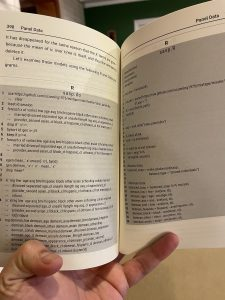# R versions of Stata foreach loops

One thing Stata users miss in R is an easy way to do the following operation.

foreach x of varlist lnw age asq bmi hispanic black other asian schooling cohab married divorced //
separated age_cl unsafe llength reg asq_cl appearance_cl provider_second asian_cl black_cl hispanic_cl // othrace_cl hot massage_cl {
egen mean ‘x’=mean(‘x’), by(id)
_
gen demean ‘x’=‘x’ – mean ‘x’

drop mean*
}

This example is taken from Scott Cunningham’s superbook, Causal Inference: the Mixtape. In the lower right of the picture you can see the demeaned variables being created one by one. So how does one perform an operation to create a large number of variables?  My preferred method is to take advantage of the .SD functionality in data.table. Create a character vector like this: myvars <- c(“lnw”,”age”) with all the variables you want.

demean <- function(x) x- mean(x)
DM <- DT[,lapply(.SD,demean),by=id,.SDcols=myvars]

Note how general this is since demean could be replaced a great number of other operations.

Also note that DM won’t have all the “demean_lnw” variables. The demeaned variables in DM keep their names from before. i think that’s a plus for various reasons.  if you want the variable names, Grant McDermott showed me that you just use the := as follows:

DT[, paste0(myvars, ‘_demean’) := lapply(.SD,demean), .SDcols=myvars, by=id]

However suppose you want to do something more along the lines of a foreach loop. then the following is more like the Stata code in terms of style and effects:

for(i in 1:length(myvars)) {
DT <- within(DT,{assign(paste0(“demean_”,myvars[i]),get(myvars[i])-ave(get(myvars[i]),id))})
}

The crucial function to learn is assign. Its first argument is string to be used as the name of the variable you’ll create and the second argument is the value you want to assign to that variabel. The get function just converts a string like “age” into an R object age.